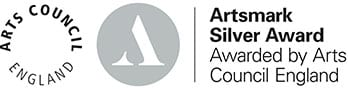# Mathematics

## Mathematics

### Course content, structure and method of assessment

A level Mathematics provides a framework within which a large number of young people continue the subject beyond GCSE level. It supports their mathematical needs across a broad range of other subjects at this level and provides a basis for subsequent quantitative work in a very wide range of higher education courses and in employment. It also supports the study of A level Further Mathematics.

A level Mathematics builds from GCSE Mathematics and introduces calculus and its applications. It emphasises how mathematical ideas are interconnected and how mathematics can be applied to model situations mathematically using algebra and other representations, to help make sense of data, to understand the physical world and to solve problems in a variety of contexts, including social sciences and business. It prepares students for further study and employment in a wide range of disciplines involving the use of mathematics.

### Why study A level mathematics?

If you wish to follow:

• An Engineering course at university
• A Maths related degree
• Any Medical course
• Any Science related course
• Accountancy/Economics

### Method of assessment

Assessment is by three exams, each of 2 hours in duration.

• Paper 1: (100 marks): content; pure mathematics: proof, algebra, calculus, exponentials and logarithms, geometry; trigonometry and numerical methods are examinable.
• Paper 2: (100 marks; two sections 50 marks each): content may include any from paper 1 and mechanics content
• Paper 3: (100 marks; two sections 50 marks each) – content may include any from paper 1 and statistical content including; sampling, data presentation, probability, distributions and including; vectors, kinematics, Newton’s laws on forces and moments. Hypothesis testing.

Level: A level

Board: AQA